Win up to 100% scholarship on Aakash BYJU'S JEE/NEET courses with ABNAT Win up to 100% scholarship on Aakash BYJU'S JEE/NEET courses with ABNAT

# JEE Main 2022 June 28 – Shift 1 Maths Question Paper with Solutions

The JEE Main 2022 June 28 – Shift 1 Maths Question Paper with Solutions are provided on this page. The JEE Main 2022 answer keys are prepared by experts at BYJU’S. Students are recommended to revise and learn the JEE Main 2022 question paper solutions to score better marks in the JEE Main exams. Practising these solutions will definitely improve the confidence of the students. Find the JEE Main 2022 June 28 – Shift 1 Maths Question Paper with Solutions below.

## JEE Main 2022 June 28th Maths Shift 1 Question Paper and Solutions

#### SECTION – A

Multiple Choice Questions: This section contains 20 multiple choice questions. Each question has 4 choices (1), (2), (3) and (4), out of which ONLY ONE is correct.

1. If

$$\begin{array}{l}\displaystyle\sum\limits_{k=1}^{31}\left ( ^{31}C_k \right )\left ( ^{31}C_{k-1} \right )-\displaystyle\sum\limits_{k=1}^{30}\left ( ^{30}C_k \right )\left ( ^{30}C_{k-1} \right )=\frac{\alpha\left ( 60! \right )}{\left ( 30! \right )\left ( 31! \right )}\end{array}$$
where α ∈ R, then the value of 16α is equal to

(A) 1411

(B) 1320

(C) 1615

(D) 1855

Sol.

$$\begin{array}{l}\displaystyle\sum\limits_{k=1}^{31}~^{31}C_k~^{31}C_{k-1}-\displaystyle\sum\limits_{k=1}^{30}~^{30}C_k~^{30}C_{k-1}\end{array}$$
$$\begin{array}{l}=\displaystyle\sum\limits_{k=1}^{31}~^{31}C_k\cdot^{31}C_{32-k}-\displaystyle\sum\limits_{k=1}^{30}~^{30}C_k\cdot^{30}C_{31-k}\end{array}$$

= 62C3260C31

$$\begin{array}{l}=\frac{60!}{31!29!}\left ( \frac{62.61}{32.30}-1 \right ) =\frac{60!}{31!29!}\frac{2822}{32.30}\end{array}$$
$$\begin{array}{l}\alpha=\frac{2822}{32}\end{array}$$
⇒ 16α = 1411

2. Let a function ƒ : N →N be defined by

$$\begin{array}{l}f\left ( n \right )=\left[\begin{matrix}2n & n=2,~4,~6,~8,\dots\\n-1, & n=3,~7,~11,~15,\dots \\\frac{n+1}{2}, & n=1,~5,~9,~13,\dots \\\end{matrix}\right. \end{array}$$
then, ƒ is

(A) One-one but not onto

(B) Onto but not one-one

(C) Neither one-one nor onto

(D) One-one and onto

Sol. When n = 1, 5, 9, 13 then (n + 1)/2 will give all odd numbers.

When n = 3, 7, 11, 15 …

n – 1 will be even but not divisible by 4

When n = 2, 4, 6, 8, …

Then 2n will give all multiples of 4

So range will be N.

And no two values of n give same y, so function is one-one and onto.

3. If the system of linear equations

2x + 3yz = –2

x + y + z = 4

xy + |λ|z = 4λ – 4

where λ∈ R, has no solution, then

(A) λ = 7

(B) λ = –7

(C) λ = 8

(D) λ2 = 1

Sol.

$$\begin{array}{l}\Delta=\begin{vmatrix}2 & 3 & -1 \\1 & 1 & 1 \\1 & -1 & \left|\lambda \right| \\\end{vmatrix}=0 \end{array}$$
⇒ |λ| = 7

But at λ = 7, Dx = Dy = Dz = 0

P1 : 2x + 3yz = –2

P2 :x + y + z = 4

P3 :xy + |λ|z = 4λ – 4

So clearly, 5P2 – 2P1 = P3, so at λ = 7, system of equation is having infinite solutions.

So λ = –7 is correct answer.

4. Let A be a matrix of order 3 × 3 and det (A) = 2. Then det (det (A) adj (5 adj (A3))) is equal to ______.

(A) 512 × 106

(B) 256 × 106

(C) 1024 × 106

(D) 256 × 1011

Sol. |A| = 2

= 253⋅ 23⋅ |A3|4

= 253⋅ 23⋅ 212 = 106⋅ 512

5. The total number of 5-digit numbers, formed by using the digits 1, 2, 3, 5, 6, 7 without repetition, which are multiple of 6, is

(A) 36

(B) 48

(C) 60

(D) 72

Sol. Number should be divisible by 6 and it should be even.

Total sum = 1 + 2 + 3 + 5 + 6 + 7 = 24

So number removed should be of type 3.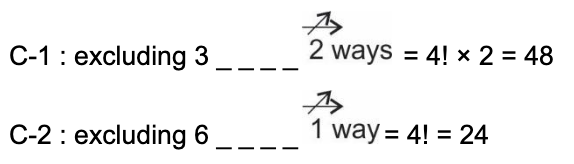Total cases = 48 + 24 = 72

6. Let A1, A2, A3, … be an increasing geometric progression of positive real numbers. If A1A3A5A7 = 1/1296 and A2 + A4 = 7/36 then, the value of A6 + A8 + A10 is equal to

(A) 33

(B) 37

(C) 43

(D) 47

Sol.

$$\begin{array}{l}\frac{A_4}{r^3}\cdot\frac{A_4}{r}\cdot A_4r\cdot A_4r^3=\frac{1}{1296}\end{array}$$
$$\begin{array}{l}A_4=\frac{1}{6} \end{array}$$
$$\begin{array}{l}A_2=\frac{7}{36}-\frac{1}{6}=\frac{1}{36}\end{array}$$

So A6 + A8 + A10 = 1 + 6 + 36

= 43

7. Let [t] denote the greatest integer less than or equal to t. Then, the value of the integral

$$\begin{array}{l}\displaystyle\int\limits_0^1\left [-8x^2+6x-1 \right ]dx \end{array}$$
is equal to

(A) –1

$$\begin{array}{l}(B)\ \frac{-5}{4}\end{array}$$
$$\begin{array}{l}(C)\ \frac{\sqrt{17}-13}{8} \end{array}$$
$$\begin{array}{l}(D)\ \frac{\sqrt{17}-16}{8} \end{array}$$

Sol.

$$\begin{array}{l}\displaystyle\int\limits_0^1\left [ -8x^2+6x-1 \right ]dx \end{array}$$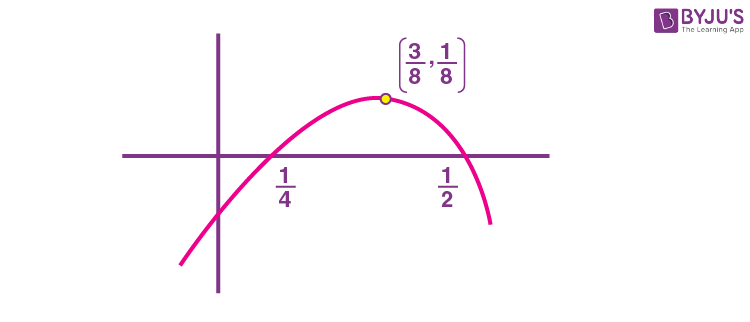$$\begin{array}{l}=\displaystyle\int\limits_0^{\frac{1}{4}}\left ( -1 \right )dx+\displaystyle\int\limits_{\frac{1}{4}}^{\frac{3}{4}}0dx+\displaystyle\int\limits_{\frac{1}{2}}^{\frac{3}{4}}-1dx+\displaystyle\int\limits_{\frac{3}{4}}^{\frac{3+\sqrt{17}}{8}}-2dx+\displaystyle\int\limits_{\frac{3+\sqrt{17}}{18}}^{1}-3dx\end{array}$$
$$\begin{array}{l}=-\frac{1}{4}-\frac{1}{4}-2\left ( \frac{3+\sqrt{17}}{8}-\frac{3}{4} \right )-3\left ( 1-\frac{3+\sqrt{17}}{8} \right )\end{array}$$
$$\begin{array}{l}=\frac{\sqrt{17}-13}{8}\end{array}$$

8. Let f: ℝ → ℝ be defined as

$$\begin{array}{l}f\left( x \right )=\left[\begin{matrix}\left [ e^x \right ], & x<0 \\ae^x+\left [ x-1 \right ], & 0\leq x<1 \\b+\left [ \sin\left ( \pi x \right ) \right ],&1\leq x<2 \\\left [ e^{-x} \right ]-c, & x\geq 2 \\\end{matrix}\right.\end{array}$$

Where a, b, c ∈  ℝ and [t] denotes greatest integer less than or equal to t. Then, which of the following statements is true?

(A) There exists a, b, c ∈  ℝ  such that ƒiscontinuous on ∈  ℝ .

(B) If ƒ is discontinuous at exactly one point, then a + b + c = 1

(C) If ƒ is discontinuous at exactly one point, then a + b + c ≠ 1

(D) ƒ is discontinuous at atleast two points, for any values of a, b and c

Sol.

$$\begin{array}{l}f\left ( x \right )\left\{\begin{matrix}0 & x<0 \\ae^x-1 & 0\leq x<1 \\b & x = 1 \\b-1 & 1<x<2 \\-c & x\geq 2 \\\end{matrix}\right.\end{array}$$

To be continuous at x = 0

a – 1 = 0

to be continuous at x = 1

ae – 1 = b = b – 1 ⇒ not possible

to be continuous at x = 2

b – 1 = – cb + c = 1

If a = 1 and b + c = 1 then f(x) is discontinuous at exactly one point.

9. The area of the region

$$\begin{array}{l}\left\{\left ( x,y \right ):y^2\leq 8x,y\geq \sqrt{2}x,x\geq 1 \right\}\end{array}$$
is

$$\begin{array}{l}(A)\ \frac{13\sqrt{2}}{6}\end{array}$$
$$\begin{array}{l}(B)\ \frac{11\sqrt{2}}{6}\end{array}$$
$$\begin{array}{l}(C)\ \frac{5\sqrt{2}}{6}\end{array}$$
$$\begin{array}{l}(D)\ \frac{19\sqrt{2}}{6}\end{array}$$

Sol.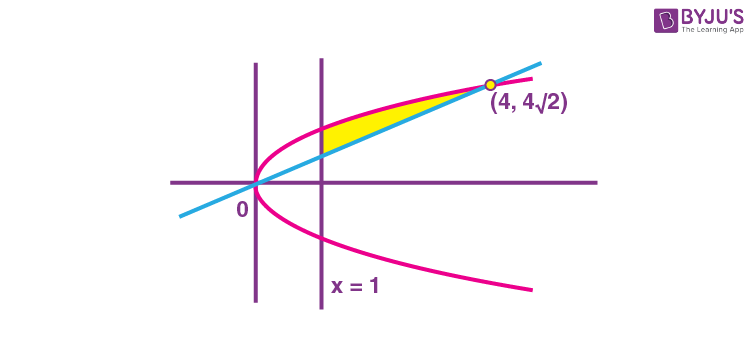Required area

$$\begin{array}{l}=\displaystyle\int\limits_1^4\left ( \sqrt{8x}-\sqrt{2}x \right )dx\end{array}$$
$$\begin{array}{l}\left.=\frac{2\sqrt{8}}{3}x^\frac{3}{2}-\frac{x^2}{\sqrt{2}} \right|_1^4\end{array}$$
$$\begin{array}{l}=\frac{16\sqrt{3}}{3}-\frac{16}{\sqrt{2}}-\frac{2\sqrt{8}}{3}+\frac{1}{\sqrt{2}}\end{array}$$
$$\begin{array}{l}=\frac{11\sqrt{2}}{6}\ sq.units\end{array}$$

10. Let the solution curve y = y(x) of the differential equation

$$\begin{array}{l}\left [\frac{x}{\sqrt{x^2-y^2}}+e^\frac{y}{x} \right ]x\frac{dy}{dx}=x+\left [\frac{x}{\sqrt{x^2-y^2}}+e^\frac{y}{x} \right ]y\end{array}$$
pass through the points (1, 0) and (2α, α), α> 0. Then α is equal to

$$\begin{array}{l}(A)\ \frac{1}{2}\textup{exp}\left ( \frac{\pi}{6}+\sqrt{e}-1 \right )\end{array}$$
$$\begin{array}{l}(B) \frac{1}{2}\textup{exp}\left ( \frac{\pi}{3}+e-1 \right )\end{array}$$
$$\begin{array}{l}(C)\ \textup{exp}\left ( \frac{\pi}{6}+\sqrt{e}+1 \right )\end{array}$$
$$\begin{array}{l}(D)\ 2~\textup{exp}\left ( \frac{\pi}{3}+\sqrt{e}-1 \right )\end{array}$$

Sol.

$$\begin{array}{l}\left (\frac{1}{\sqrt{1-\frac{y^2}{x^2}}}+e^\frac{y}{x} \right )\frac{dy}{dx}=1+\left ( \frac{1}{\sqrt{1-\frac{y^2}{x^2}}}+e^\frac{y}{x} \right )\frac{y}{x}\end{array}$$

Putting y = tx

$$\begin{array}{l}\left ( \frac{1}{\sqrt{1-t^2}}+e^t \right )\left ( t+x\frac{dt}{dx} \right )=1+\left ( \frac{1}{\sqrt{1-t^2}}+e^t \right )t\end{array}$$
$$\begin{array}{l} \Rightarrow x\left ( \frac{1}{\sqrt{1-t^2}}+e^t \right )\frac{dt}{dx}=1\end{array}$$

⇒ sin–1t + et = lnx + C

$$\begin{array}{l}\Rightarrow \sin^{-1}\left ( \frac{y}{x} \right )+e^{y/x}=\textup{In}~x+C\end{array}$$

atx = 1, y = 0

So, 0 + e0 = 0 + CC = 1

at (2α, α)

$$\begin{array}{l}\sin^{-1}\left ( \frac{y}{x} \right )+e^{y/x}=\textup{In}~x+1\end{array}$$
$$\begin{array}{l}\Rightarrow \frac{\pi}{6}+e^\frac{1}{2}-1=\textup{In}\left ( 2\alpha \right )\end{array}$$
$$\begin{array}{l}\Rightarrow \alpha=\frac{1}{2}e^{\left ( \frac{\pi}{6}+e^\frac{1}{2}-1 \right )}\end{array}$$

11. Let y = y(x) be the solution of the differential equation

$$\begin{array}{l}x\left ( 1-x^2 \right )\frac{dy}{dx}+\left ( 3x^2y-y-4x^3 \right )=0,~x>1\end{array}$$
with y(2) = –2. Then y(3) is equal to

(A) –18

(B) –12

(C) –6

(D) –3

Sol.

$$\begin{array}{l}\frac{dy}{dx}+\frac{y(3x^2-1)}{x\left ( 1-x^2 \right )}=\frac{4x^3}{x\left ( 1-x^2 \right )}\end{array}$$
$$\begin{array}{l}IF = e^{\int\frac{3x^2-1}{x-x^3}dx}=e^{\textup-{\textup{In}\left|x^3-x \right|}}=e^{-\textup{In}\left ( x^3-x \right )}\end{array}$$
$$\begin{array}{l}=\frac{1}{x^3-x}\end{array}$$

Solution of D.E. can be given by

$$\begin{array}{l}y.\frac{1}{x^3-x}=\int\frac{4x^3}{x\left ( 1-x^2 \right )}\cdot\frac{1}{x\left ( x^2-1 \right )}dx\end{array}$$
$$\begin{array}{l}\Rightarrow \frac{y}{x^3-x}=\int\frac{-4x}{\left ( x^2-1 \right )^2}dx\end{array}$$
$$\begin{array}{l}\Rightarrow \frac{y}{x^3-x}=\frac{2}{\left ( x^2-1 \right )} +c\end{array}$$

at x = 2, y = –2

$$\begin{array}{l}\frac{-2}{6}=\frac{2}{3}+c~~\Rightarrow~c=-1\end{array}$$
$$\begin{array}{l}\textup{at}~x=3\Rightarrow \frac{y}{24}=\frac{2}{8}-1\Rightarrow y=-18\end{array}$$

12. The number of real solutions of x7 + 5x3 + 3x + 1 = 0 is equal to ______.

(A) 0

(B) 1

(C) 3

(D) 5

Sol.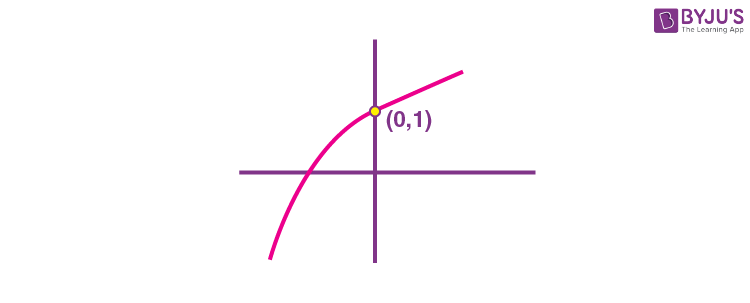$$\begin{array}{l}f’\left ( x \right )=7x^6+15x^2+3>0~\forall x\in R\end{array}$$

f(x) is always increasing.

So clearly, it intersects the x-axis at only one point.

13. Let the eccentricity of the hyperbola

$$\begin{array}{l}H:\frac{x^2}{a^2}-\frac{y^2}{b^2}=1\end{array}$$
be √(5/2) and length of its latus rectum be 6√2, If y = 2x + c is a tangent to the hyperbola H. then the value of c2 is equal to

(A) 18

(B) 20

(C) 24

(D) 32

Sol.

$$\begin{array}{l}1+\frac{b^2}{a^2}=\frac{5}{2}\Rightarrow \frac{b^2}{a^2}=\frac{3}{2}\end{array}$$
$$\begin{array}{l}\frac{2b^2}{a}=6\sqrt{2}\Rightarrow 2\cdot\frac{3}{2}\cdot a=6\sqrt{2}\end{array}$$
$$\begin{array}{l}\Rightarrow a=2\sqrt{2},b^2=12\end{array}$$
$$\begin{array}{l}c^2=a^2m^2-b^2=8.4-12=20\end{array}$$

14. If the tangents drawn at the points O(0, 0) and P(1 + √5, 2) on the circle x2 + y2 – 2x – 4y = 0 intersect at the point Q, then the area of the triangle OPQ is equal to

$$\begin{array}{l}(A)\ \frac{3+\sqrt{5}}{2}\end{array}$$
$$\begin{array}{l}(B)\ \frac{4+2\sqrt{5}}{2}\end{array}$$
$$\begin{array}{l}(C)\ \frac{5+3\sqrt{5}}{2}\end{array}$$
$$\begin{array}{l}(D)\ \frac{7+3\sqrt{5}}{2}\end{array}$$

Sol.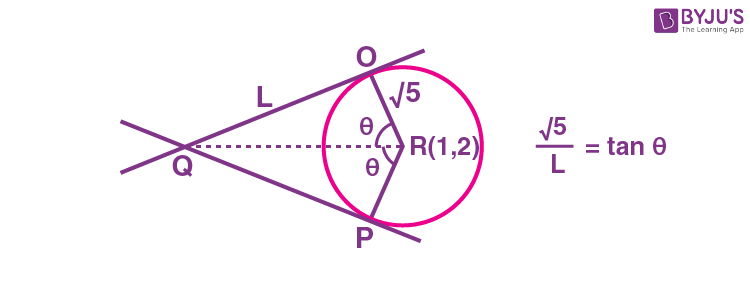$$\begin{array}{l}\tan~2\theta=2\Rightarrow \frac{2\tan\theta}{1-\tan^2\theta} =2\end{array}$$
$$\begin{array}{l}\tan~\theta=\frac{\sqrt{5}-1}{2}\end{array}$$
(as θ is acute)

$$\begin{array}{l}\textup{Area}=\frac{1}{2}L^2\sin 2\theta=\frac{1}{2}\cdot\frac{5}{\tan^2\theta}\cdot2\sin\theta\cos\theta \end{array}$$
$$\begin{array}{l}=\frac{5\sin\theta\cos\theta}{\sin^2\theta}\cdot\cos^2\theta\end{array}$$

= 5cot θ.cos2θ

$$\begin{array}{l}=5.\frac{2}{\sqrt{5}-1}\cdot\frac{1}{1+\left ( \frac{\sqrt{5}-1}{2} \right )^2}\end{array}$$
$$\begin{array}{l}=\frac{10}{\sqrt{5}-1}\cdot\frac{4}{4+6-2\sqrt{5}} \end{array}$$
$$\begin{array}{l}=\frac{40}{2\sqrt{5}\left ( \sqrt{5}-1 \right )^2}=\frac{4\sqrt{5}}{6-2\sqrt{5}}\end{array}$$
$$\begin{array}{l}=\frac{4\sqrt{5}\left ( 6+2\sqrt{5} \right )}{16} \end{array}$$
$$\begin{array}{l}=\frac{\sqrt{5}\left ( 3+\sqrt{5} \right )}{2} \end{array}$$

15. If two distinct points Q, R lie on the line of intersection of the planes –x + 2y – z = 0 and 3x – 5y + 2z = 0 and

$$\begin{array}{l}PQ=PR=\sqrt{18}\end{array}$$
where the point P is (1, –2, 3), then the area of the triangle PQR is equal to

$$\begin{array}{l}(A)\ \frac{2}{3}\sqrt{38}\end{array}$$
$$\begin{array}{l}(B)\ \frac{4}{3}\sqrt{38}\end{array}$$
$$\begin{array}{l}(C)\ \frac{8}{3}\sqrt{38} \end{array}$$
$$\begin{array}{l}(D)\ \sqrt{\frac{152}{3}} \end{array}$$

Sol.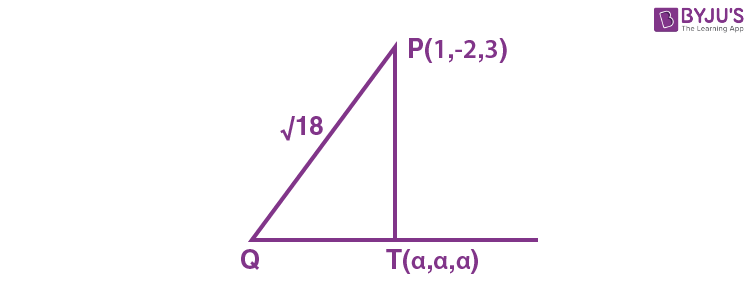Line L is x = y = z

$$\begin{array}{l}\overrightarrow{PQ}.\left ( \hat{i}+\hat{j}+\hat{k} \right )=0\end{array}$$

⇒ (α – 3) + α + 2 + α – 1 = 0

$$\begin{array}{l}\Rightarrow \alpha=\frac{2}{3}\end{array}$$
so,
$$\begin{array}{l}T=\left ( \frac{2}{3},\frac{2}{3},\frac{2}{3} \right )\end{array}$$
$$\begin{array}{l}PT=\sqrt{\frac{38}{3}} \end{array}$$
$$\begin{array}{l}\Rightarrow QT=\frac{4}{\sqrt{3}}\end{array}$$

So, Area

$$\begin{array}{l}=\left ( \frac{1}{2}\times\frac{4}{\sqrt{3}}\times\frac{\sqrt{38}}{\sqrt{3}} \right )\cdot2\end{array}$$
$$\begin{array}{l}=\frac{4\sqrt{38}}{3}~\textup{sq~units}\end{array}$$

16. The acute angle between the planes P1 and P2, when P1 and P2 are the planes passing through the intersection of the planes 5x + 8y + 13z – 29 = 0 and 8x – 7y + z – 20 = 0 and the points (2, 1, 3) and (0, 1, 2), respectively, is

$$\begin{array}{l}(A)\ \frac{\pi}{3} \end{array}$$
$$\begin{array}{l}(B)\ \frac{\pi}{4} \end{array}$$
$$\begin{array}{l}(C)\ \frac{\pi}{6} \end{array}$$
$$\begin{array}{l}(D)\ \frac{\pi}{12} \end{array}$$

Sol. Family of Plane’s equation can be given by

(5 + 8λ)x + (8 – 7λ)y + (13 + λ) z – (29 + 20λ) = 0

P1 passes through (2, 1, 3)

⇒ (10 + 16λ) + (8 – 7λ) + (39 + 3λ) – (29 + 20λ) = 0

⇒ –8λ + 28 = 0

$$\begin{array}{l}\Rightarrow \lambda=\frac{7}{2}\end{array}$$

d.r,s of normal to P1

$$\begin{array}{l}\left<33, \frac{-33}{2},\frac{33}{2} \right>\textup{or}\left<1,-\frac{1}{2},\frac{1}{2} \right>\end{array}$$

P2 passes through (0, 1, 2)

• 8 – 7λ + 26 + 2λ – (29 + 20λ) = 0

5 – 25λ = 0

$$\begin{array}{l}\Rightarrow \lambda=\frac{1}{5} \end{array}$$

d.r, s of normal to P2

$$\begin{array}{l}\left<\frac{33}{5},\frac{33}{5},\frac{66}{5} \right>\textup{or}~\left<1,1,2 \right>\end{array}$$

Angle between normals

$$\begin{array}{l}=\frac{\left ( \hat{i}-\frac{1}{2}\hat{j}+\frac{1}{2}\hat{k} \right )\cdot\left ( \hat{i}+\hat{j}+2\hat{k} \right )}{\frac{\sqrt{3}}{2}~~~~~~~~~~~~~\sqrt{6}}\end{array}$$
$$\begin{array}{l}\cos\theta=\frac{1-\frac{1}{2}+1}{3}=\frac{1}{2}\end{array}$$
$$\begin{array}{l}\theta=\frac{\pi}{3}\end{array}$$

17. Let the plane

$$\begin{array}{l}P:\vec{r}\cdot\vec{a}=d \end{array}$$
contain the line of intersection of two planes
$$\begin{array}{l}\vec{r}.\left ( \hat{i}+3\hat{j}-\hat{k} \right )=6\end{array}$$
and
$$\begin{array}{l}\vec{r}\cdot\left ( -6\hat{i}+5\hat{j}-\hat{k} \right )=7\end{array}$$
. If the plane P passes through the point (2, 3, 1/2),
$$\begin{array}{l}\text{then the value of}\ \frac{\left|13\vec{a} \right|^2}{d^2}\ \text{is equal to}\end{array}$$

(A) 90

(B) 93

(C) 95

(D) 97

Sol. P1: x + 3yz = 6

P2: –6x + 5yz = 7

Family of planes passing through line of intersection of P1 and P2 is given by x(1 – 6λ) + y(3 + 5λ) + z (–1 – λ) – (6 + 7λ) = 0

It passes through (2, 3, 1/2)

So,

$$\begin{array}{l}2\left ( 1-6\lambda \right )+3\left ( 3+5\lambda \right )+\frac{1}{2}\left ( -1-\lambda \right )-\left ( 6+7\lambda \right )=0\end{array}$$
$$\begin{array}{l}\Rightarrow 2-12\lambda+9+15\lambda-\frac{1}{2}-\frac{\lambda}{2}-6-7\lambda=0 \end{array}$$
$$\begin{array}{l}\Rightarrow \frac{9}{2}-\frac{9\lambda}{2}=0\Rightarrow \lambda=1 \end{array}$$

Required plane is

–5x + 8y – 2z – 13 = 0

Or

$$\begin{array}{l}\vec{r}\cdot\left ( -5\hat{i}+8\hat{j}-2\hat{k} \right ) =13\end{array}$$
$$\begin{array}{l}\frac{\left|13\vec{a} \right|^2}{\left|d \right|^2}=\frac{13^2}{\left ( 13 \right )^2}\cdot\left|\vec{a} \right|^2=93\end{array}$$

18. The probability, that in a randomly selected 3-digit number at least two digits are odd, is

$$\begin{array}{l}(A)\ \frac{19}{36} \end{array}$$
$$\begin{array}{l}(B)\ \frac{15}{36} \end{array}$$
$$\begin{array}{l}(C)\ \frac{13}{36} \end{array}$$
$$\begin{array}{l}(D)\ \frac{23}{36} \end{array}$$

Sol. Required cases = Total – all digits even – exactly one digit even

Total = 900 ways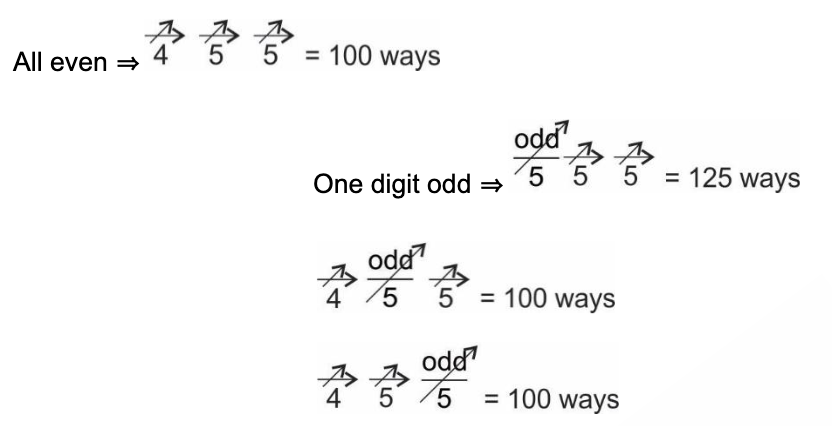$$\begin{array}{l}\text{Required probability} =\frac{900-425}{900}=\frac{19}{36} \end{array}$$

19. Let AB and PQ be two vertical poles, 160 m apart from each other. Let C be the middle point of B and Q, which are feet of these two poles. Let π/8 and θ be the angles of elevation from C to P and A, respectively. If the height of pole PQ is twice the height of pole AB, then tan2θ is equal to

$$\begin{array}{l}(A)\ \frac{3-2\sqrt{2}}{2}\end{array}$$
$$\begin{array}{l}(B)\ \frac{3+\sqrt{2}}{2}\end{array}$$
$$\begin{array}{l}(C)\ \frac{3-2\sqrt{2}}{4}\end{array}$$
$$\begin{array}{l}(D)\ \frac{3-\sqrt{2}}{4}\end{array}$$

Sol.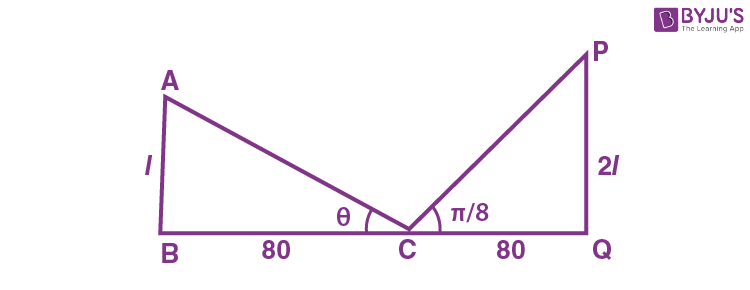$$\begin{array}{l}\frac{\ell}{80} =\tan\theta….(i)\end{array}$$
$$\begin{array}{l}\frac{2\ell}{80} =\tan\frac{\pi}{8}….(ii)\end{array}$$

From (i) and (ii)

$$\begin{array}{l}\frac{1}{2}=\frac{\tan\theta}{\tan\frac{\pi}{8}} \Rightarrow \tan^2\theta=\frac{1}{4}\tan^2\frac{\pi}{8} \end{array}$$
$$\begin{array}{l}\Rightarrow \tan^2\theta=\frac{\sqrt{2}-1}{4\left ( \sqrt{2}+1 \right )}=\frac{3-2\sqrt{2}}{4} \end{array}$$

20. Let p, q, r be three logical statements. Consider the compound statements

S1 : ((~p) ∨q) ∨ ((~p) ∨r) and

S2 :p→ (qr)

Then, which of the following is NOT true?

(A) If S2 is True, then S1 is True

(B) If S2is False, then S1 is False

(C) If S2 is False, then S1 is True

(D) If S1 is False, then S2 is False

Sol. S1: (~pq) ∨ (~ pr)

≅ (~ pqr)

S2: ~p ∨ (qr)

Both are same

So, option (C) is incorrect.

#### SECTION – B

Numerical Value Type Questions: This section contains 10 questions. In Section B, attempt any five questions out of 10. The answer to each question is a NUMERICAL VALUE. For each question, enter the correct numerical value (in decimal notation, truncated/rounded-off to the second decimal place; e.g. 06.25, 07.00, –00.33, –00.30, 30.27, –27.30) using the mouse and the on-screen virtual numeric keypad in the place designated to enter the answer.

1. Let R1 and R2 be relations on the set {1, 2, ….., 50} such that R1 ={(p, pn) :p is a prime and n≥ 0 is an integer} and R2 = {(p, pn) : p is a prime and n = 0 or 1}. Then, the number of elements in R1R2 is ______.

Sol. R1R2 = {(2, 22), (2, 23), (2, 24), (2, 25), (3, 32)

(3, 33), (5, 52), (7, 72)}

So number of elements = 8

2. The number of real solutions of the equation is _____.

Sol. Dividing by e2x

e2x + 4ex – 58 + 4ex + e–2x = 0

⇒ (ex + ex)2 + 4(ex + ex) – 60 = 0

Let ex + ex= t∈[2, ∞)

t2 + 4t – 60 = 0

t = 6 is only possible solution

ex + ex = 6 ⇒e2x – 6ex + 1 = 0

Let ex = p,

p2 – 6p + 1 = 0

$$\begin{array}{l}\Rightarrow p=\frac{3+\sqrt{5}}{2}~\textup{OR}~\frac{3-\sqrt{5}}{2} \end{array}$$

So

$$\begin{array}{l}x=\textup{ln}\left (\frac{3+\sqrt{5}}{2} \right )~\textup{OR}~\textup{ln}\left (\frac{3-\sqrt{5}}{2} \right ) \end{array}$$

3. The mean and standard deviation of 15 observations are found to be 8 and 3, respectively. On rechecking, it was found that, in the observations, 20 was misread as 5. Then, the correct variance is equal to _______.

Sol.

$$\begin{array}{l}\frac{\Sigma x_i^2}{15}-8^2=9\Rightarrow \Sigma x_i^2=15\times73=1095 \end{array}$$
$$\begin{array}{l}\text{Let}\ \overline{x}_c\ \text{be corrected mean}\ \overline{x}_c=9\end{array}$$

∑xc21095 – 25 + 400 = 1470

Correctvariance

$$\begin{array}{l}=\frac{1470}{15}-\left ( 9 \right )^2=98-81=17\end{array}$$

4. If

$$\begin{array}{l}\vec{a}=2\hat{i}+\hat{j}+3\hat{k},\vec{b}=3\hat{i}+3\hat{j}+\hat{k}\ \text{and}\ \vec{c}=c_1\hat{i}+c_2\hat{j}+c_3\hat{k}\end{array}$$
are coplanar vectors and
$$\begin{array}{l}\vec{a}\cdot\vec{c}=5,\vec{b}\perp\vec{c}\end{array}$$
then 122(c1 + c2 + c3) is equal to _________.

Sol. 2C1 + C2 + 3C3 = 5 …(i)

3C1 + 3C2 + C3 = 0 …(ii)

$$\begin{array}{l}\left [\vec{a}\vec{b}\vec{c} \right ] =\begin{vmatrix}2 & 1 & 3 \\3 & 3 & 1 \\C_1 & C_2 & C_3 \\\end{vmatrix}\end{array}$$

= 2(3C3C2) – 1(3C3C1) + 3(3C2 – 3C1)

= 3C3 + 7C2 – 8C1

⇒ 8C1 – 7C2 – 3C3 = 0 …(iii)

$$\begin{array}{l}C_1=\frac{10}{122},C_2=\frac{-85}{122},C_3=\frac{225}{122} \end{array}$$

So 122(C1 + C2 + C3) = 150

5. A ray of light passing through the point P(2, 3) reflects on the x-axis at point A and the reflected ray passes through the point Q(5, 4). Let R be the point that divides the line segment AQ internally into the ratio 2 : 1. Let the co-ordinates of the foot of the perpendicular M from R on the bisector of the angle PAQ be (α, β). Then, the value of 7α + 3β is equal to ___________.

Sol.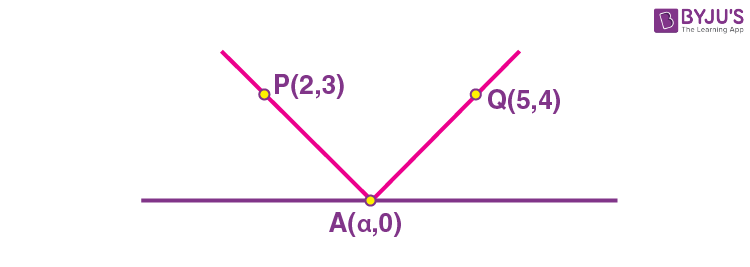$$\begin{array}{l}\frac{4}{5-\alpha}=\frac{3}{\alpha-2}\Rightarrow 4\alpha-8=15-3\alpha\end{array}$$
$$\begin{array}{l}\alpha=\frac{23}{7} \end{array}$$
$$\begin{array}{l}A=\left ( \frac{23}{7},0 \right ) Q=\left ( 5,4 \right )\end{array}$$
$$\begin{array}{l}R=\left ( \frac{10+\frac{23}{7}}{3},\frac{8}{3} \right ) \end{array}$$
$$\begin{array}{l}=\left ( \frac{31}{7},\frac{8}{3} \right ) \end{array}$$

Bisector of angle PAQ is X = 23/7.

$$\begin{array}{l}\Rightarrow M=\left ( \frac{23}{7},\frac{8}{3} \right ) \end{array}$$

So, 7α + 3β = 31

6. Let l be a line which is normal to the curve y = 2x2 + x + 2 at a point P on the curve. If the point Q(6, 4) lies on the line l and O is origin, then the area of the triangle OPQ is equal to ___________.

Sol.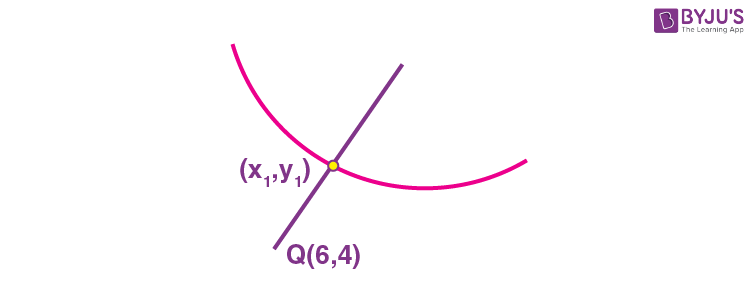$$\begin{array}{l}\frac{y_1-4}{x_1-6}=-\frac{1}{4x_1+1} \end{array}$$
$$\begin{array}{l}\Rightarrow \frac{2x_1^2+x_1-2}{x_1-6}=-\frac{1}{4x_1+1} \end{array}$$

⇒ 6 – x1 = 8x13 + 6x12 – 7x1 – 2

⇒ 8x13 + 6x12 – 6x1 – 8 = 0

So x1 = 1 ⇒y1 = 5

$$\begin{array}{l}\text{Area} =\left|\frac{1}{2}\begin{vmatrix}0 & 0 & 1 \\6 & 4 & 1 \\1 & 5 & 1 \\\end{vmatrix} \right|=13\end{array}$$

7. Let A = {1, a1, a2a18, 77} be a set of integers with 1 <a1<a2<….<a18< 77. Let the set A + A = {x + y :x, yA} contain exactly 39 elements. Then, the value of a1 + a2 +…+a18 is equal to _____.

Sol. If we write the elements of A + A, we can certainly find 39 distinct elements as 1 + 1, 1 + a1, 1 + a2,…..1 + a18, 1 + 77, a1 + 77, a2 + 77,……a18 + 77, 77 + 77.

It means all other sums are already present in these 39 values, which is only possible in case when all numbers are in A.P.

Let the common difference be ‘d’.

77 = 1 + 19d ⇒d = 4

So,

$$\begin{array}{l}\displaystyle\sum\limits_{i=1}^{18}a_1=\frac{18}{2}\left [2a_1+17d \right ]=9\left [10+68 \right ]=702\end{array}$$

8. The number of positive integers k such that the constant term in the binomial expansion of

$$\begin{array}{l}\left ( 2x^3+\frac{3}{x^k} \right )^{12},x\neq0\end{array}$$
is 28 . l, where l is an odd integer, is______.

Sol.

$$\begin{array}{l}T_{r+1}=^{12}C_r\left ( 2x^3 \right )^{12-r}\left ( \frac{3}{x^k} \right )^r\end{array}$$
$$\begin{array}{l}=^{12}C_r2^{12-r}3^r~~X^{36-3r-kr}\end{array}$$

For constant term 36 – 3rkr = 0

$$\begin{array}{l}r = \frac{36}{3+k} \end{array}$$

So, k can be 1, 3, 6, 9, 15, 33

In order to get 28, check by putting values of k and corresponding in general term. By checking, it is possible only where k = 3 or 6

9. The number of elements in the set

$$\begin{array}{l}\left\{z=a+ib~\epsilon~\mathbb{C}:a,b~\epsilon~\mathbb{Z}~\textup{and}~1<\left|z-3+2i \right|<4 \right\}\end{array}$$
is __________.

Sol.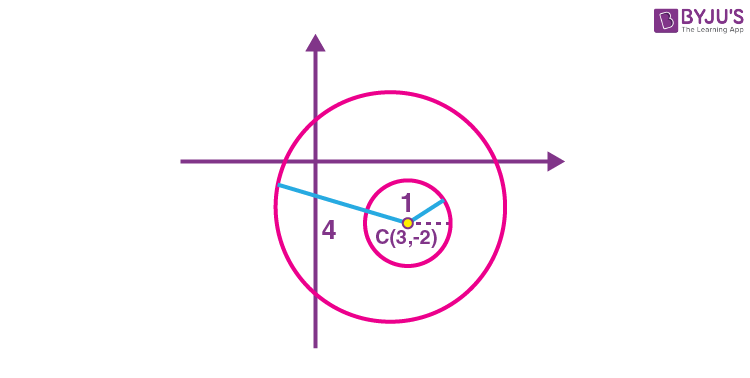at line y = –2, we have (5, –2) (6, –2) (1, –2) (0, –2) ⇒ 4 points

at line y = –1, we have (4, –1) (5, –1) (6, –1) (2, –1) (1, –1) (0, –1) ⇒ 6 points

at line y = 0, we have (0, 0) (1, 0) (2, 0) (3, 0) (4, 0) (5, 0) (6, 0) ⇒ 7 points

at line y = 1, we have (1, 1), (2, 1), (3, 1), (4, 1), (5, 1) i.e. 5 points

symmetrically

at line y = –5, we have 5 points

at line y = –4, we have 7 points

at line y = –3, we have 6 points

So Total integral points = 2(5 + 7 + 6) + 4

= 40

10. Let the lines

$$\begin{array}{l}y+2x=\sqrt{11}+7\sqrt{7}\ \text{and}\ 2y+x=2\sqrt{11}+6\sqrt{7}\end{array}$$
be normal to a circle C : (xh)2 + (yk)2 = r2. If the line
$$\begin{array}{l}\sqrt{11}y-3x=\frac{5\sqrt{17}}{3}+11 \end{array}$$
is tangent to the circle C, then the value of (5h – 8k)2 + 5r2 is equal to _______.

Sol. L1 : y + 2x = √11 + 7√7

L2 : 2y + x = 2√11 + 6√7

Point of intersection of these two lines is centre of circle i.e.

$$\begin{array}{l}\left ( \frac{8}{3}\sqrt{7},\sqrt{11}+\frac{5}{3}\sqrt{7} \right ) \end{array}$$
$$\begin{array}{l}\text{Perpendicular from centre to line}\ 3x-\sqrt{11}y+\left ( \frac{5\sqrt{77}}{3}+11 \right )=0\end{array}$$

$$\begin{array}{l}\Rightarrow r=\left|\frac{8\sqrt{7}-11-\frac{5}{3}\sqrt{77}+\frac{5\sqrt{77}}{3}+11}{\sqrt{20}} \right| \end{array}$$
$$\begin{array}{l}=\left|\sqrt{\frac{7}{5}} \right|=\sqrt{\frac{7}{5}}\textup{units}\end{array}$$

So (5h – 8K)2 + 5r2

$$\begin{array}{l}=\left ( \frac{40}{3}\sqrt{7}-8\sqrt{11}-\frac{40}{3}\sqrt{7} \right )^2+5.16.\frac{7}{5}\end{array}$$

= 64 × 11 + 112 = 816.

### Download PDF of JEE Main 2022 June 28 Shift 1 Maths Paper & Solutions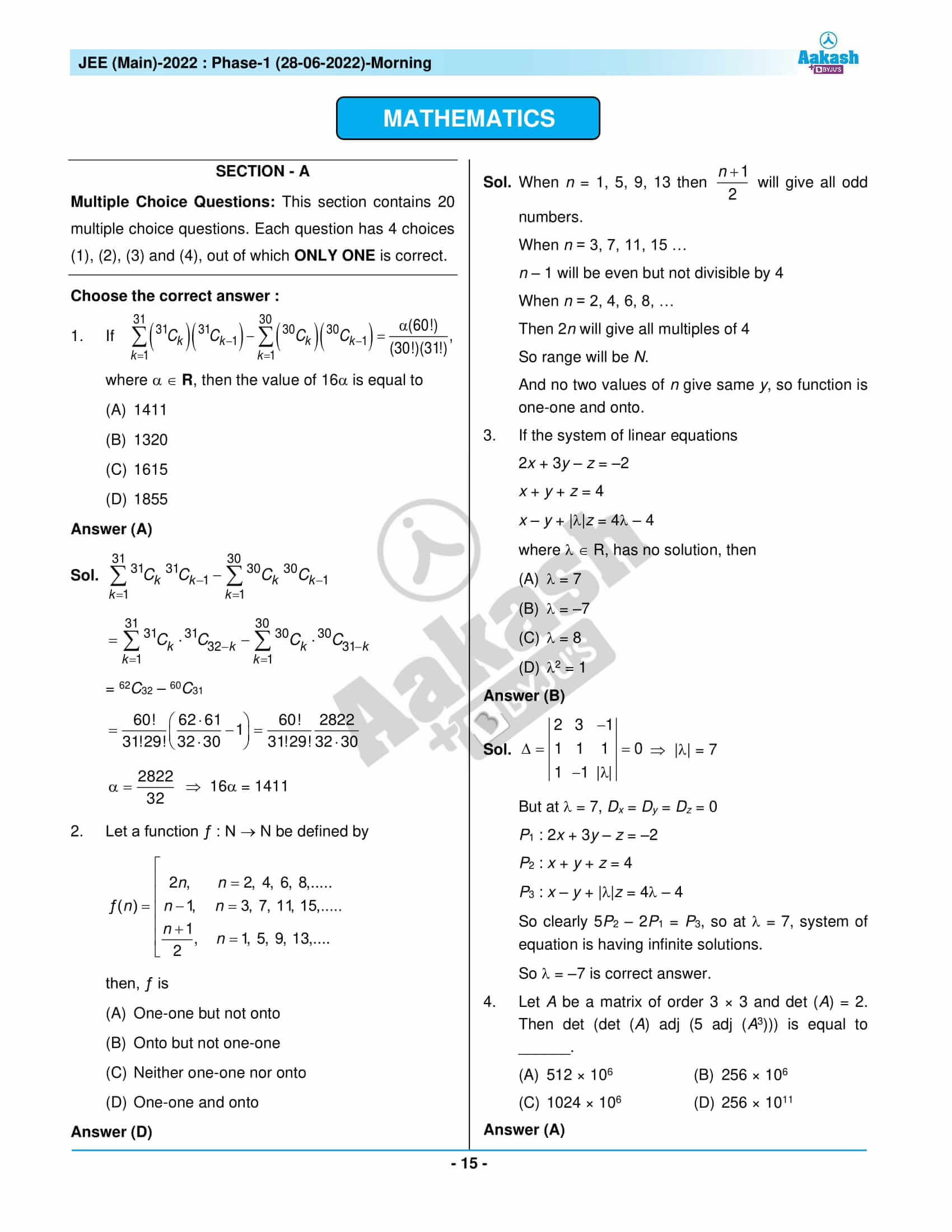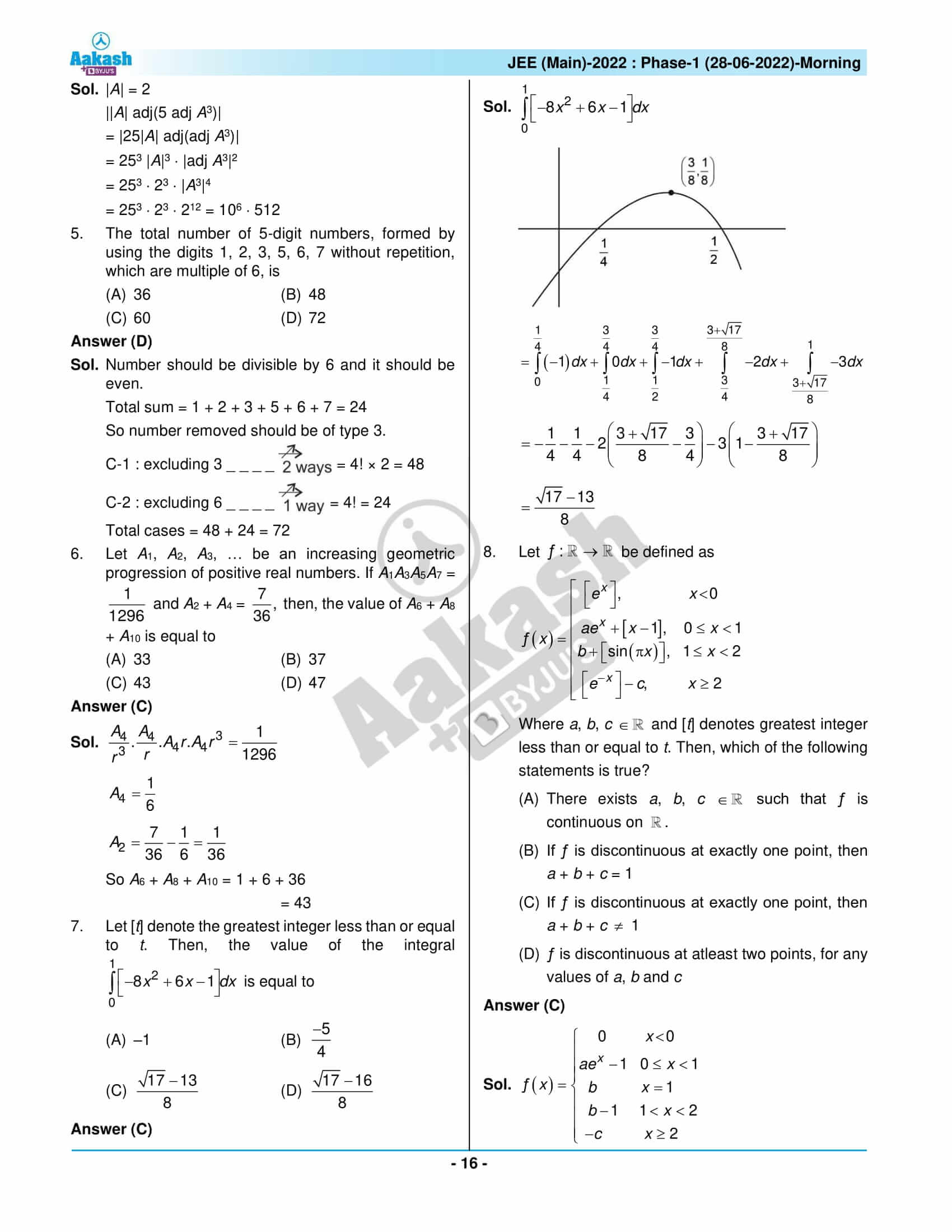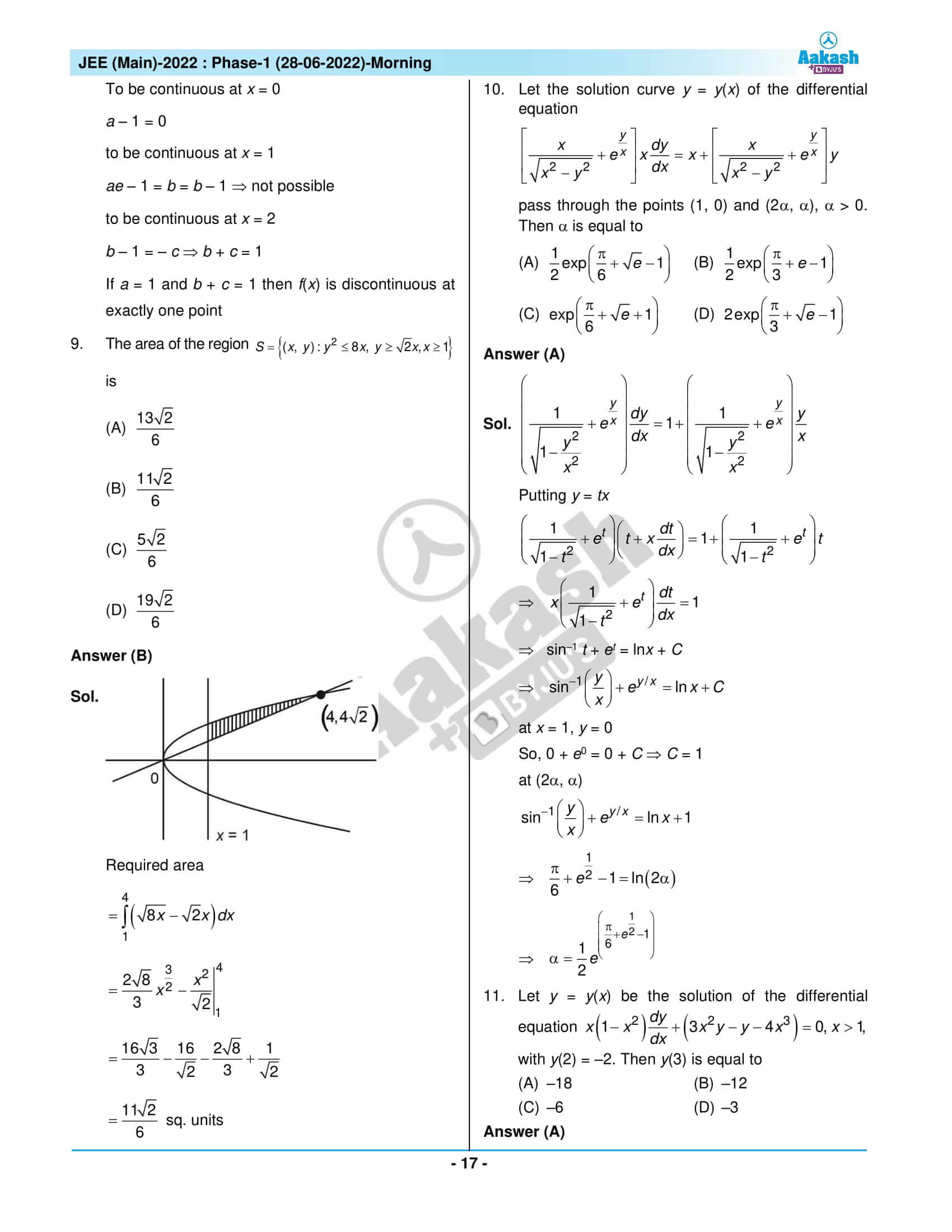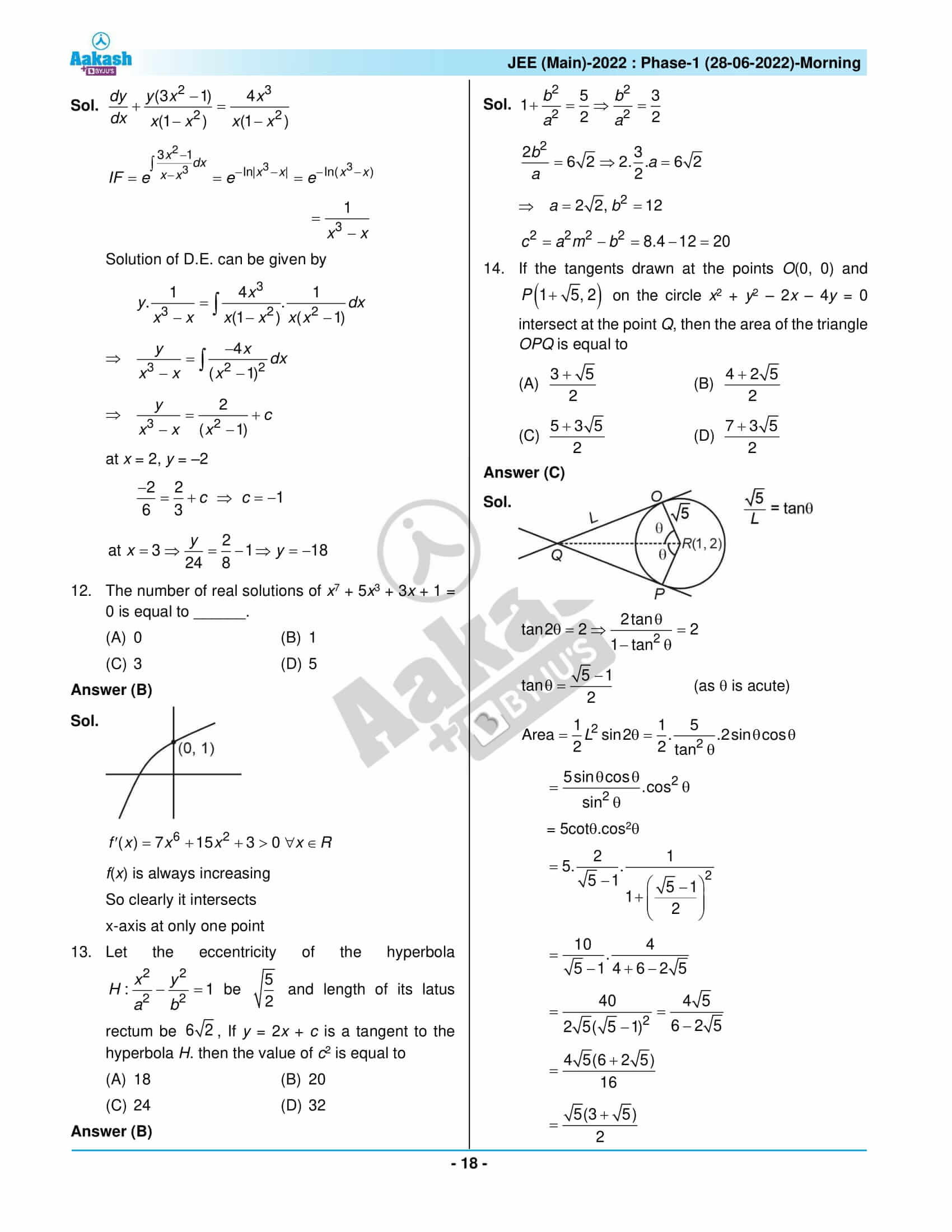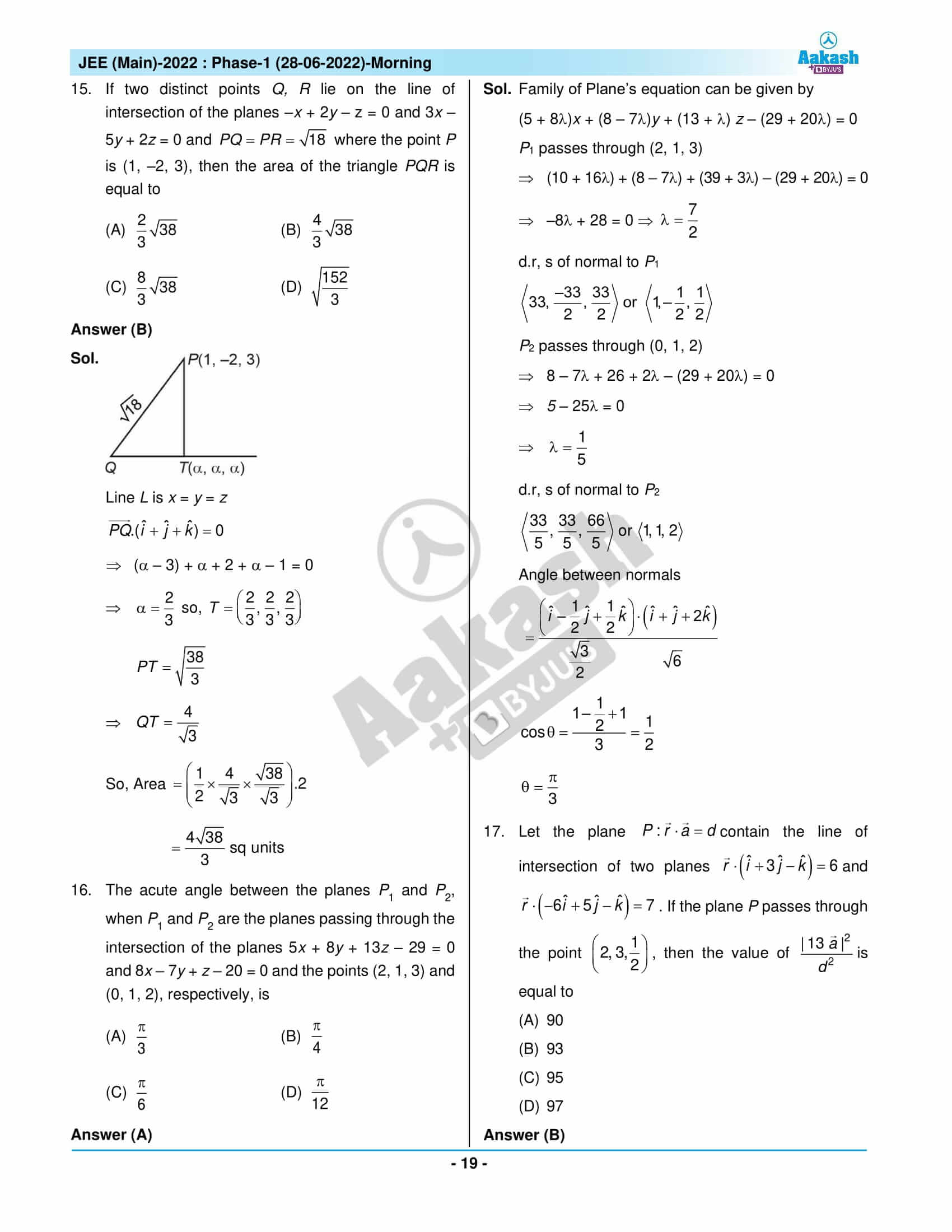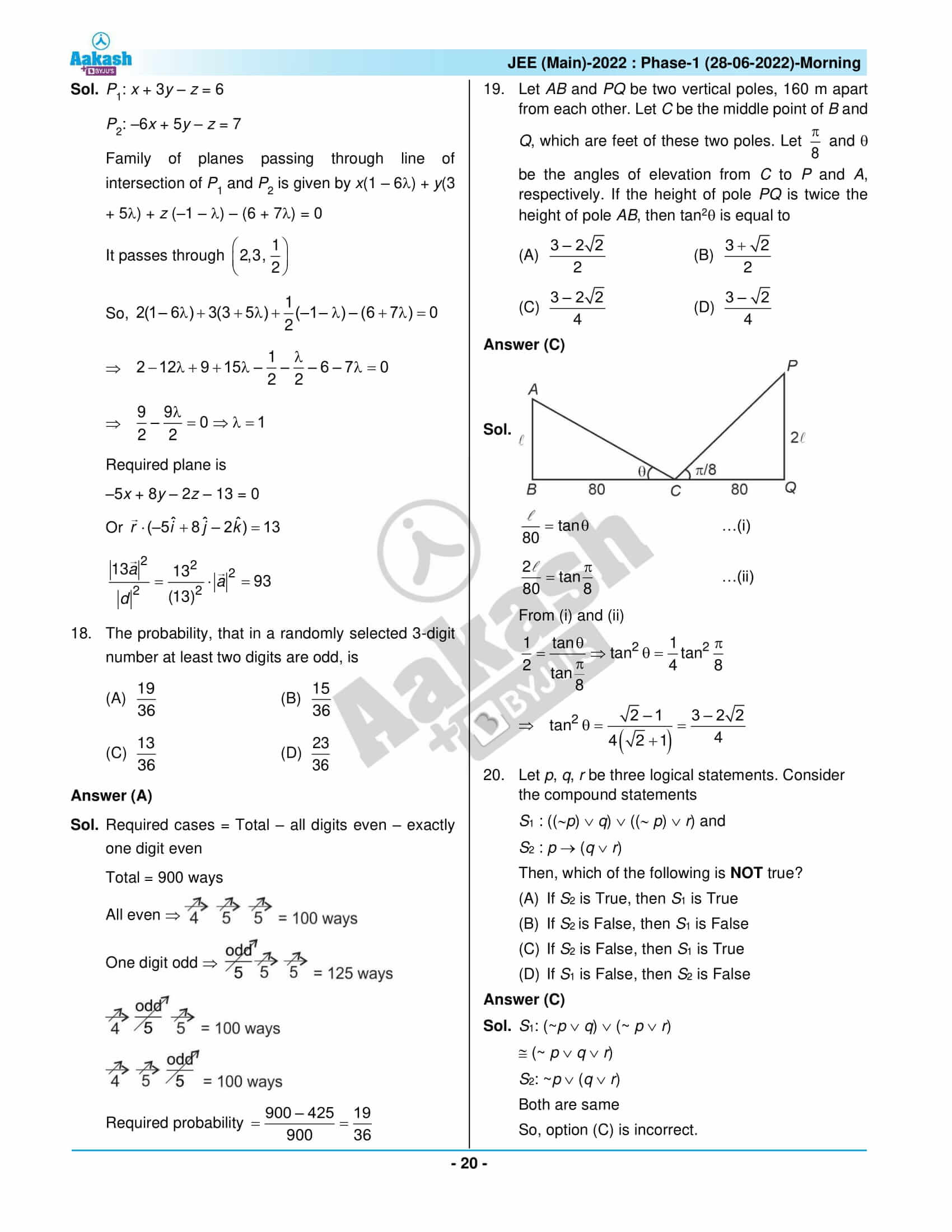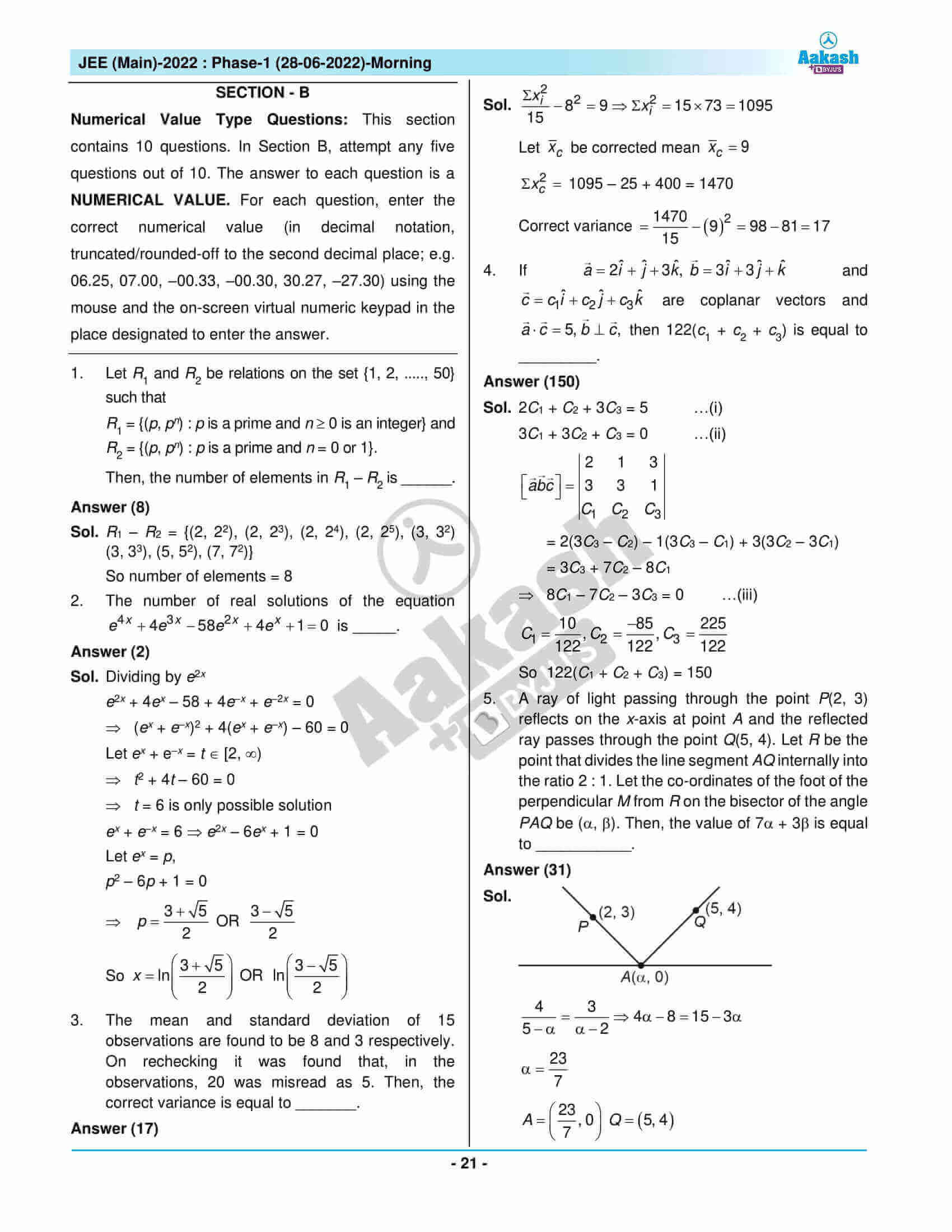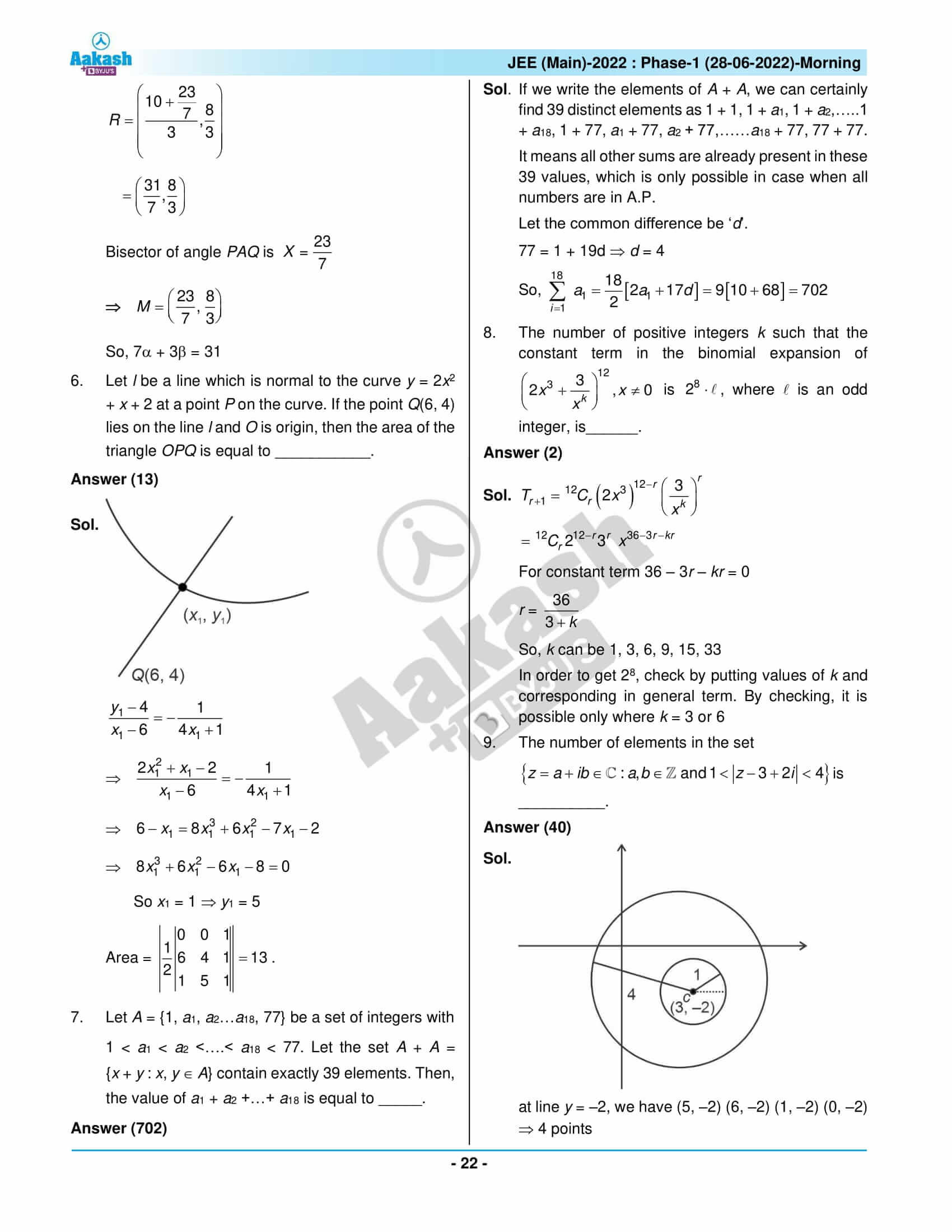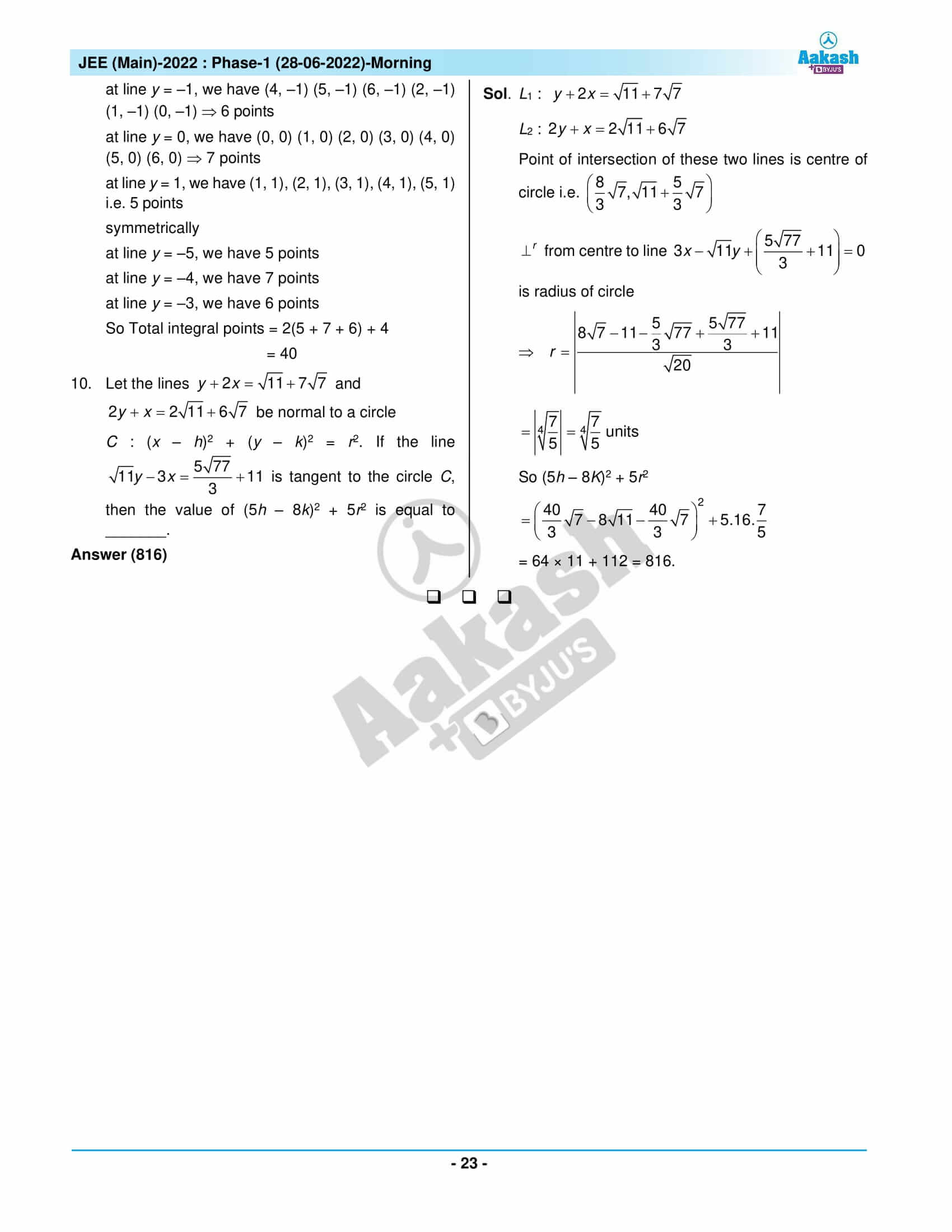## JEE Main 2022 June 28th Shift 1 Question Paper & Solutions

### JEE Main 2022 June 28 Shift 1 Question Paper – Physics Solutions### JEE Main 2022 June 28 Shift 1 Question Paper – Chemistry Solutions### JEE Main 2022 June 28 Shift 1 Question Paper – Maths Solutions## Frequently Asked Questions – FAQs

Q1

### How many questions are there in JEE Main 2022 June 28 Shift 1 Maths question paper?

There are 30 questions in JEE Main 2022 June 28 Shift 1 Maths question paper. 20 questions are in section A and 10 are in section B.

Q2

### How many questions should students need to attempt in section B of the JEE Main 2022 June 28 Maths Shift 1 question paper?

Students need to attempt only 5 out of 10 questions in section B of the JEE Main 2022 June 28 Maths Shift 1 question paper.

Q3

### How was the JEE Main 2022 Maths June 28 Shift 1 question paper?

The JEE Main 2022 Maths June 28 Shift 1 question paper was of moderate difficulty. It had 5 easy questions, 13 medium questions and 1 difficult question as per the memory-based question paper.

Q4

### What is the difficulty level of questions asked from Binomial theorem in JEE Main 2022 June 28 Maths Shift 1 question paper?

The questions from the Binomial theorem were medium difficult questions.

Q5

### What is the overall difficulty level of JEE Main 2022 June 28 Shift 1 Maths question paper?

The overall difficulty level of JEE Main 2022 June 28 Shift 1 Maths question paper was 1.79 out of 3.